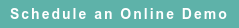# RoyaltyStat Blog

### Posts by Topic

A practice in transfer pricing is to select a “net profit indicator” (such as operating profit margin or return on assets) and calculate quartiles among i = 1, 2, …, N selected enterprise-level comparables. This practice produces unreliable profit indicators that can’t survive audit scrutiny. A major fallacy of this practice is to estimate structural equations when reduced-forms should be estimated. In this blog, we discuss and solve this fallacy.

#### Indirect Least Squares (ILS)

Define enterprise-level S = net sales, C = total costs (XOPR = COGS + XSGA), and P = operating profits before depreciation and amortization (OIBDP). All three variables are measured in the reported country currency such as USD or EUR per year. A typical operating profit equation calculated in transfer pricing is an old Buridan-Oresme linear proportion:

(1)  P(i) = β S(i) computed among N comparables.

The slope coefficient (β = operating profit margin) is expected to be positive but less than one. One interpretation is that P(i) is impetus, the slope coefficient is the weight (molecular mass), and S(i) is velocity (prime mover) of the enterprise system.

Equation (1) is deterministic and lacks an intercept. To correct these specifications errors, we postulate a stochastic linear equation with an intercept:

##### (2)  P(i) = α + β S(i) + U(i)

where U(i) is a random error with mean zero and constant variance among N comparables. Other functional forms such as a power function can be tested using comparable enterprise-level (S(i) P(i)) ordered pair financials.

The slope coefficient of models (1) and (2) is unreliable because the postulated relation (subject to empirical testing) between operating profits and net sales needs to consider a simultaneous system including an accounting definition of profits:

##### (3)  S(i) = C(i) + P(i)

Equation (3) is true by definition and thus is not subject to errors.

Substitute structural equation (3) into (2) and obtain a reduced-form, which is proper to estimate the intercept and slope parameters (model (5)):

##### (5)  P(i) = λ0 + λ1 C(i) + V(i)

where the intercept = λ0 = α / (1 – β), slope = λ= β / (1 – β), and V(i) = U(i) / (1 – β).

The reduced-form of a structural equation system such as (2) and (3) is an alternative method of writing the model or system such that each endogenous variable is expressed as a function of the exogenous variable alone. In (2) and (3) above, total costs are the exogenous variable and net sales and operating profits are endogenous (determined by an equation in the system). Structural equations describe a major component of the system, such as profits equal net sales minus total costs (3), and profits are proportional to net sales in the same period (2).

We get more reliable estimates of arm's length net profit margin using operating profit before depreciation and amortization as the dependent variable (P = OIBDP = EBITDA instead of P = OIADP = EBIT), and excluding depreciation of gross property, plant & equipment (PPEGT) and amortization of acquired intangibles (DP) from total costs (C = COGS + XSGA) as the independent variable. One important reason is that DP includes amortization of acquired intangibles (AM), which may be irrelevant to the controlled economic activities (functions performed) being tested for arm’s length behavior. Another reason is that differences in gross asset vintages and depreciation rates make operating profit after depreciation and amortization less homogeneous among comparables.

It follows from (5) that a reliable measure of profit margin is the indirect slope estimate:

##### (6)  β = λ1 / (1 + λ1)

which is known as indirect least squares. See among others: Arthur Goldberger, Introductory Econometrics, Harvard University Press, 1998, Chapter 20 (Estimation of simultaneous-equation models), pp. 220-222.

We have not found the standard error (SE) of (6) in several econometrics textbooks consulted, but we calculate it using a formula derived from the Delta (Dorfman's approximation for the variance of a random variable) method:

##### (7)  SE(β) = SE(λ1) / (1 + λ1)2

The parameter estimates λ1 and SE(λ1) are results of running the reduced-form regression equation (5). The Delta method of estimating standard errors is described in Bradley Efron, The Jackknife, the Bootstrap, and Other Resampling Plans, SIAM (Society for Industrial and Applied Mathematics), 1982, Chapter 6.5, pp. 42-44.

#### Policy Implication

Using structural form (1) or (2) produces inconsistent (unreliable) estimates of β (profit margin). A consistent estimator has a probability limit of the sample statistics equal to the parameter that it purports to estimate. See https://en.wikipedia.org/wiki/Consistent_estimator

Structural equation estimates are not consistent, and we must rely on reduced-form estimates to compute reliable net profit margins and their standard errors. Like computing Kahn-Kalecki-Keynes’ investment multiplier, equation (5) results from a simultaneous profit determination system that includes accounting and behavioral equations. Instead of using inconsistent profit model (1) or (2), it's more defensible running linear regression (5) to compute consistent MNE arm’s length profit margins with formula (6) and calculate their standard errors using (7).

Else we can obtain a better regression fit running other reduced-form functional types, such as running an alternative power function or double-log regression model of operating profits vs. total costs.

Published on Oct 27, 2018 8:45:49 AM

Ednaldo Silva (Ph.D.) is founder and managing director of RoyaltyStat. He helped draft the US transfer pricing regulations and developed the comparable profits method called TNNM by the OECD. He can be contacted at: esilva@royaltystat.com

RoyaltyStat provides premier online databases of royalty rates extracted from unredacted license agreements
and normalized company financials (income statement, balance sheet, cash flow). We provide high-quality data, built-in analytical tools, customer training and attentive technical support.Topics: Net Profit Indicator